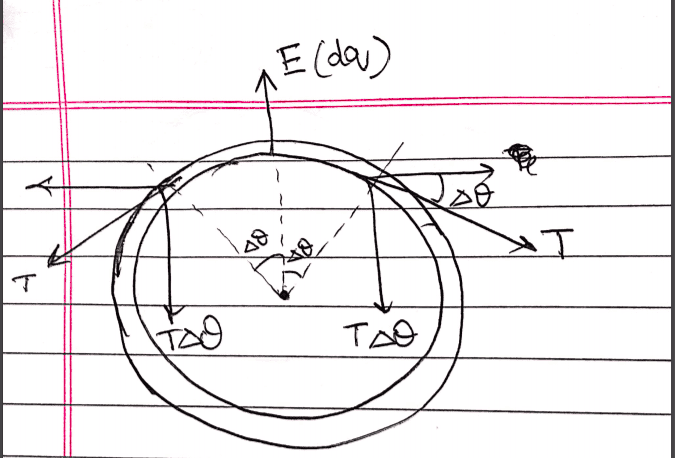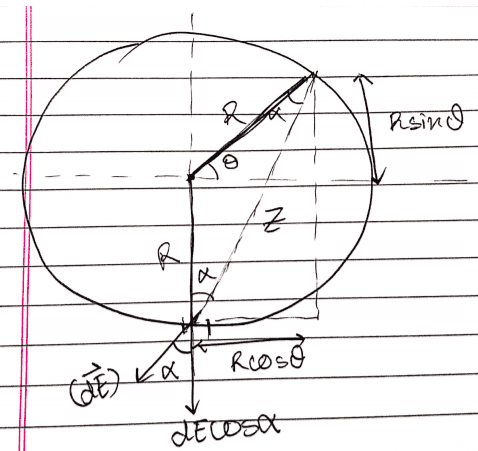# Tension developed in a charged ring

Hamiltonian
Homework Statement:
calculate the tension developed in ring of radius ##R##( of negligible thickness) and charge ##Q##
Relevant Equations:
-consider a small element that subtends an angle ##2\Delta \theta## at the center of the ring. balancing the forces on this element gives:
(let the field due to the ring be at its circumference be ##E##).
$$2T\Delta \theta = E(dq) = E (\frac{Q}{2\pi})(2\Delta \theta)$$
$$T = \frac{EQ}{2\pi}$$
now the problem is reduced to finding the field due to the charged ring at its circumference:let the distance from an infinitesimal charge ##(dq)## to the required point on the circumference be ##z##
$$z^2 = (Rsin\theta + R)^2 + (Rcos\theta)^2 = 2R^2(1+sin\theta)$$
$$(dE) = k(dq)/z^2 = \frac {k(dq)}{2R^2(1+sin\theta)}$$
by symmetry arguments the net field at the circumference will be only in the y direction:
$$(dE_{net}) = (dE)(cos\alpha)$$
$$\alpha = \pi/4 - \theta/2$$
$$(dq) = (Q/2\pi) d\theta$$
$$dE_{net} = k(dq)\frac{cos(\pi/4 - \theta/2)}{1+sin\theta} = \frac{kQ}{4\pi R^2} \frac{cos(\pi/4 - \theta/2)}{1+sin\theta} (d\theta)$$
$$E_{net} = \frac{kQ}{4\pi R^2} \int_0^{2\pi} \frac{cos(\pi/4 - \theta/2)}{1+sin\theta} d\theta$$

I put the integral into wolframalpha and it does not converge!
that would mean the field is infinite which obviously can't be true.
is there any physical significance to this or have i made a mistake in calculating the field at the circumference of the ring.

I had also tried to find the field at any general point in the plane of the ring and then wanted to find the field at circumference but the integral proved to be very complex (wolframalpha exceeded standard computation time) hence i didn't go down that path.